# RBSE Maths Class 10 Chapter 9: Coordinate Geometry Important Questions and Solutions

RBSE Class 10 Maths Chapter 9 – Coordinate Geometry Important questions and solutions are given here. All these questions provided are as per the new guidelines of the board to help the students for their exams. The RBSE Class 10 important questions and solutions available at BYJU’S contain all the concepts, which are important from the examination point of view.

Chapter 9 of RBSE Class 10 has two exercises which contain questions of finding distance between two points, midpoints and so on. Different formulas are available in this chapter, such as distance between two points, section formula for internal and external division. These concepts are covered here, along with the questions and solutions.

### RBSE Maths Chapter 9: Exercise 9.1 Textbook Important Questions and Solutions

Question 1: Find the coordinates of points P, Q, R, and S from the given figure.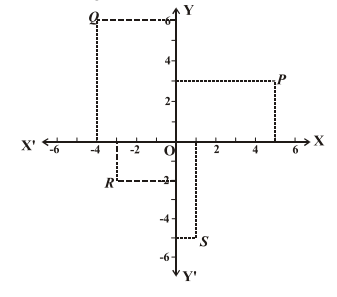Solution:

Coordinates of point P = (5, 3)

Coordinates of point Q = (-4, 6)

Coordinates of point R = (-3, -2)

Coordinates of point S = (1, -5)

Question 2: Plot the points of the following coordinates.

(1, 2), (-1, 3), (-2, -4), (3, -2), (2, 0), (0, 3)

Solution:

Let us plot the coordinates of given points as shown below.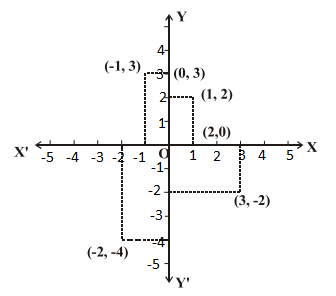Question 3: By taking rectangular coordinates axis, plot the points O(0, 0), P(3, 0) and R(0, 4). If OPQR is rectangle, then find coordinates of Q.

Solution:

To plot the given points, first draw two perpendicular axes XOX’ and YOY’ and plot them as shown below.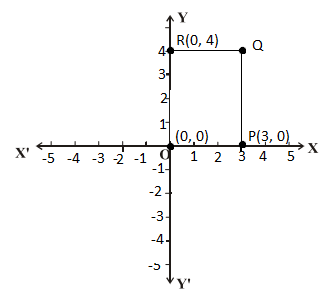Given that, OPQR is a rectangle.

From the graph, point Q is at a distance of 3 units from O on the X axis and at a distance of 4 units from O on the Y axis.

Thus, for point Q, abscissa is 3 and ordinate is 4.

Therefore, the coordinates of point Q = (3, 4)

Question 4: Plot the points (-1, 0), (1, 0), (1, 1), (0, 2), (-1, 1). Which figure is obtained, by joining them serially?

Solution:

Given points are:

(-1, 0), (1, 0), (1, 1), (0, 2), (-1, 1)

Plot these points as shown in figure given below.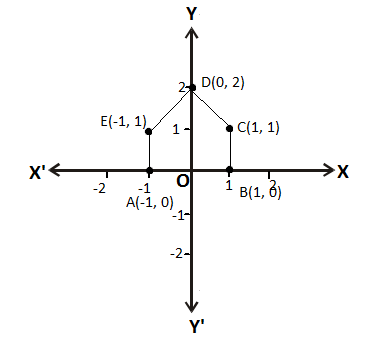Hence, the obtained figure is a pentagon.

Question 5: Find the distance between the points (-1, -1) and (8, -2).

Solution:

Distance formula to find the distance between two points (x1, y1) and (x2, y2) is:

$$\begin{array}{l}d=\sqrt{(x_2-x_1)^2+(y_2-y_1)^2}\end{array}$$

Given points are:

(x1, y1) = (-1, -1)

(x2, y2) = (8, -2)

Distance between (-1, -1) and (8, -2) is:

$$\begin{array}{l}d=\sqrt{[8-(-1)]^2+[-2-(-1)]^2}=\sqrt{(9)^2+(-1)^2}=\sqrt{81+1}=\sqrt{82}\end{array}$$

Question 6: Find the distance between the points (at12, 2at1) and (at22, 2at2).

Solution:

Distance formula to find the distance between two points (x1, y1) and (x2, y2) is:

$$\begin{array}{l}d=\sqrt{(x_2-x_1)^2+(y_2-y_1)^2}\end{array}$$

Given points are:

(x1, y1) = (at12, 2at1)

(x2, y2) = (at22, 2at2)

Distance between the points (at12, 2at1) and (at22, 2at2) is: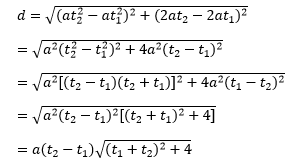Question 7: Prove that the points (2, -2), (-2, 1) and (5, 2) are vertices of a right angled triangle.

Solution:

Let the given points be:

A = (2, -2)

B = (-2, 1)

C = (5, 2)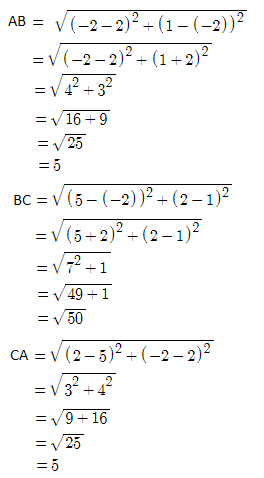AB2 + CA2 = (5)2 + (5)2

= 25 + 25

= 50

BC2 = (√50)2 = 50

BC2 = AB2 + CA2

By the converse of Pythagoras theorem, the given points are the vertices of a right angled triangle.

Hence proved.

Question 8: Prove that points (1, -2), (3, 0), (1, 2) and (-1, 0) are vertices of a square.

Solution:

Let the given points be:

A = (1, -2)

B = (3, 0)

C = (1, 2)

D = (-1, 0)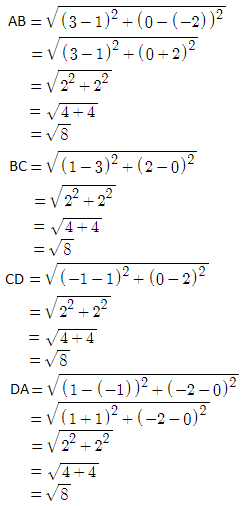Thus, AB = BC = CD = DA = √8

Diagonals AC and BD are: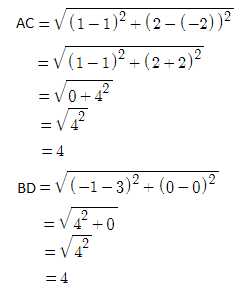Therefore, AC = BD

Hence, the given vertices form a square.

Question 9: Prove that points (1, 1), (-2, 7) and (3, -3) are collinear.

Solution:

Let the given points be:

A = (1, 1)

B = (-2, 7)

C = (3, -3)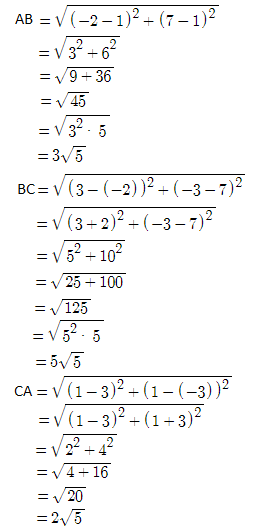AB + CA = 3√5 + 2√5 = 5√5 = BC

Therefore, BC = AB + AC

Hence, the given points are collinear.

Question 10: Find the points on the y-axis which is equidistant from points (-5, -2) and (3, 2).

Solution:

Let P(0, y) be the point on the y-axis.

Given point are:

A = (-5, -2)

B = (3, 2)

According to the given,

PA = PB

⇒ PA2 = PB2

⇒ (-5 – 0)2 + (-2 – y)2 = (3 – 0)2 + (2 – y)2

⇒ (-5)2 + (2 + y)2 = (3)2 + (2 – y)2

⇒ 25 + 4 + 4y + y2 = 9 + 4 – 4y + y2

⇒ 29 + 4y + 4y – 13 = 0

⇒ 8y + 16 = 0

⇒ 8y = -16

⇒ y = 16/8

⇒ y = -2

Therefore, the required point on the y-axis is (0, -2).

Question 11: If points (3, k) and (k, 5) are equidistant from a point (0, 2), then find the value of k.

Solution:

Let the given points be:

A = (3, k)

B = (k, 5)

P = (0, 2)

According to the given,

PA = PB

⇒ PA2 = PB2

⇒ (3 – 0)2 + (k – 2)2 = (k – 0)2 + (5 – 2)2

⇒ (3)2 + k2 – 4k + 4 = k2 + (3)2

⇒ -4k + 4 = 0

⇒ 4k = k

⇒ k = 4/4

⇒ k = 1

Question 12: If coordinates of P and Q are (a cos θ, b sin θ) and (-a sin θ, b cos θ) respectively, then show that OP2 + OQ2 = a2 + b2, where O is the origin.

Solution:

Given points are:

P = (a cos θ, b sin θ)

Q = (-a sin θ, b cos θ)

Coordinates of origin are O = (0, 0)

OP2 = (a cos θ – 0)2 + (b sin θ – 0)2

= a2 cos2θ + b2 sin2θ ….(i)

OQ2 = (-a sin θ – 0)2 + (b cos θ – 0)2

= a2 sin2θ + b2 cos2θ ….(ii)

OP2 + OQ2 = a2 cos2θ + b2 sin2θ + a2 sin2θ + b2 cos2θ

= a2(cos2θ + sin2θ) + b2(sin2θ + cos2θ)

= a2(1) + b2(1)

= a2 + b2

Therefore, OP2 + OQ2 = a2 + b2

### RBSE Maths Chapter 9: Exercise 9.2 Textbook Important Questions and Solutions

Question 13: Find the coordinates of the point which divides the line segment joining the points (3, 5) and (7, 9) in the ratio 2 : 3 internally.

Solution:

Let P(x, y) divide the line segment joining the points A(3, 5) and B(7, 9) internally in the ratio 2 : 3.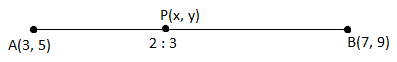Here,

(x1, y1) = (3, 5)

(x2, y2) = (7, 9)

m1 : m2 = 2 : 3

Using the formula of internal division,

x = (m1x2 + m2x1)/(m1 + m2)

= [2(7) + 3(3)]/(2 + 3)

= (14 + 9)/5

= 23/5

y = (m1y2 + m2y1)/(m1 + m2)

= [2(9) + 3(5)]/(2 + 3)

= (18 + 15)/5

= 33/5

Hence, the coordinates of the required point is (23/5, 33/5).

Question 14: Find the coordinates of the point which divides the line segment joining the points (5, -2) and (-1 1/2, 4) in the ratio 7 : 9 externally.

Solution:

Let P(x, y) divide the line segment joining the points A(5, -2) and B(-1 1/2, 4) externally in the ratio 7 : 9.

Here,

(x1, y1) = (5, -2)

(x2, y2) = (-1 ½, 4) = (-3/2, 4)

m1 : m2 = 7 : 9

Using the formula of external division,

x = (m1x2 – m2x1)/(m1 – m2)

= [7(-3/2) – 9(5) ] /(7 – 9)

= (-21/2 – 45) / (-2)

= (21 + 90) / (2 × 2)

= 111/4

y = (m1y2 – m2y1)/(m1 – m2)

= [7(4) – 9(-2)] / (7 – 9)

= (28 + 18) / (-2)

= -46/2

= -23

Hence, the coordinates of the required point is (111/4, -23).

Question 15: Prove that origin O divides the line joining the points A(1, -3) and (-3, 9) in the ratio 1 : 3 internally. Also, find the coordinates of the point which divides the line AB externally in the ratio 1 : 3.

Solution:

Let P(x, y) divide the line segment joining the points A(1, -3) and B(-3, 9) internally in the ratio 1 : 3.

Here,

(x1, y1) = (1, -3)

(x2, y2) = (-3, 9)

m1 : m2 = 1 : 3

Using the formula of internal division,

x = (m1x2 + m2x1)/(m1 + m2)

= [1(-3) + 3(1)]/(1 + 3)

= (-3 + 3)/4

= 0/4

= 0

y = (m1y2 + m2y1)/(m1 + m2)

= [1(9) + 3(-3)]/(1 + 3)

= (9 – 9)/4

= 0/5

= 0

Hence, the coordinates of the required point is (0, 0), i.e. the origin.

Let P(x, y) divide the line segment joining the points A(1, -3) and B(-3, 9) externally in the ratio 1 : 3.

Here,

(x1, y1) = (1, -3)

(x2, y2) = (-3, 9)

m1 : m2 = 1 : 3

Using the formula of external division,

x = (m1x2 – m2x1)/(m1 – m2)

= [1(-3) – 3(1) ] /(1 – 3)

= (-3 – 3) / (-2)

= (-6) / (-2)

= 3

y = (m1y2 – m2y1)/(m1 – m2)

= [1(9) – 3(-3)] / (1 – 3)

= (9 + 9) / (-2)

= -18/2

= -9

Hence, the coordinates of the required point is (3, -9).

Question 16: Find the midpoint of the line joining the points (22, 20) and (0, 16).

Solution:

Let the given points be:

(x1, y1) = (22, 20)

(x2, y2) = (0, 16)

Let P(x, y) be the midpoint of two points.

Using the midpoint formula,

x = (x1 + x2)/2

= (22 + 0)/2

= 22/2

= 11

y = (y1 + y2)/2

= (20 + 16)/2

= 36/2

= 18

Therefore, the coordinates of the mid point are (11, 18).

Question 17: In which ratio, x-axis divides the line segment which joins points (5, 3) and (-3, -2)?

Solution:

Coordinates of the point on the x-axis are (x, 0).

Let P(x, 0) divide the line segment joining the points A(5, 3) and (-3, -2) internally in the ratio m1 : m2.

Here,

(x1, y1) = (5, 3)

(x2, y2) = (-3, -2)

Using the section formula,

y-coordinate of P = (m1y2 + m2y1) / (m1 + m2)

0 = [m1(-2) + m2(3)] / (m1 + m2)

⇒ -2m1 + 3m2 = 0

⇒ 2m1 = 3m2

⇒ m1/m2 = 3/2

⇒ m1 : m2 = 3 : 2

Hence, the required ratio is 3 :2.

Question 18: In which ratio, point (11, 15) divides the line segment which joins (15, 5) and (9, 20)?

Solution:

Let P(11, 15) be the point which divides the line segment joining the points A(15, 5) and B(9, 20) internally in the ratio k : 1.

Here,

(x1, y1) = (15, 5)

(x2, y2) = (9, 20)

m1 : m2 = k : 1

Using section formula,

x-coordinate of P is

x = (m1x2 + m2x1)/(m1 + m2)

11 = [k(9) + 1(15)] / (k + 1)

⇒ 11(k + 1) = 9k + 15

⇒ 11k + 11 – 9k = 15

⇒ 2k = 15 – 11

⇒ 2k = 4

⇒ k = 4/2

⇒ k = 2

Therefore, the required ratio is 2 : 1.

Question 19: If point P(3, 5) divides the line segment which joins A(-2, 3) and B(x, y) in the ratio 4 : 7 internally, then find the coordinates of B.

Solution:

Given that, the point P(3, 5) divides the line segment joining the points A(-2, 3) and B(x, y) internally in the ratio 4 : 7.

Here,

(x1, y1) = (-2, 3)

(x2, y2) = (x, y)

m1 : m2 = 4 : 7

Using the formula of internal division,

x – coordinate of P is

= (m1x2 + m2x1)/(m1 + m2)

3 = [4(x) + 7(-2)] / (4 + 7)

3 = (4x – 14)/11

⇒ 4x – 14 = 33

⇒ 4x = 33 + 14

⇒ 4x = 47

⇒ x = 47/4

y – coordinate of P is

= (m1y2 + m2y1)/(m1 + m2)

5 = [4(y) + 7(3)] / (4 + 7)

5 = (4y + 21)/11

⇒ 4y + 21 = 55

⇒ 4y = 55 – 21

⇒ 4y = 34

⇒ y = 34/4

⇒ y = 17/2

Hence, the coordinates of the required point are (47/4, 17/2).

Question 20: Find the coordinates of point which trisects the line joining point (11, 9) and (1, 2).

Solution:

Let P(x1 y1) and Q(x2, y2) be the points which trisect the line segment joining the points A(11, 9) and B(1, 2).

Let AP = PQ = BQ = k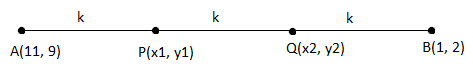PB = k + k = 2k

AQ = k + k = 2k

AP/PB = k/2k = 1/2

AP : PB = 1 : 2

AQ/BQ = 2k/k = 2/1

AQ : BQ = 2 : 1

Therefore, point P divides the line segment AB in the ratio 1 : 2 and point Q divides the line segment AB in the ratio 2 : 1.

Using the section formula for point P,

m1 : m2 = 1 : 2

x1 = [1(1) + 2(12)] / (1 + 2)

= (1 + 22)/3

= 23/3

y1 = [1(2) + 2(9)] / (1 + 2)

= (2 + 18)/3

= 20/3

Hence, the coordinates of the point P are (23/3, 20/3).

Using the section formula for point Q,

m1 : m2 = 2 : 1

x2 =[2(1) + 1(11)] / (2 + 1)

= (2 + 11)/3

= 13/3

y2 = [2(2) + 1(9)] / (2 + 1)

= (4 + 9)/3

= 13/3

Hence, the coordinates of the point Q are (13/3, 13/3).

Question 21: Find the coordinates of the point which quartersects the line joining points (-4, 0) and (0, 6).

Solution:

Let P, Q and R be the points which quartesects the line segment joining the points A(-4, 0) and B(0, 6)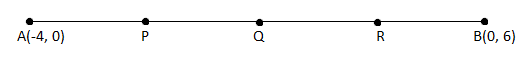.

AP = PQ = QR = RB

Q is the midpoint of AB.

Using the midpoint formula,

(x, y) = [(x1 + x2)/2, (y1 + y2)/2]

Coordinates of Q = [(-4 + 0)/2, (0 + 6)/2)

= (-4/2, 6/2)

= (-2, 3)

Thus, Q = (-2, 3)

P is the midpoint of AQ.

Coordinates of P = [(-4 – 2)/2, (0 + 3)/2]

= (-6/2, 3/2)

= (-3, 3/2)

Thus, P = (-3, 3/2)

R is the midpoint of QB.

Coordinates of R = [(-2 + 0)/2, (3 + 6)/2)

= (-2/2, 9/2)

= (-1, 9/2)

Thus, R = (-1, 9/2)

Hence, the coordinates of required points are P(-3, 3/2), Q(-2, 3) and R(-1, 9/2).

Question 22: Find the ratio in which line 3x + y = 9 divides the line segment which joins points (1, 3) and (2, 7).

Solution:

Let k : 1 be the ratio in which the line 3x + y = 9 divides the line segment joining the points A(1, 3) and B(2, 7) internally at point P(x, y).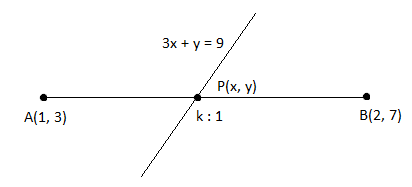Here,

(x1, y1) = (1, 3)

(x2, y2) = (2, 7)

m1 : m2 = k : 1

Using the formula of internal division,

x – coordinate of P is

= (m1x2 + m2x1)/(m1 + m2)

= [k(2) + 1(1)]/(k + 1)

= (2k + 1)/(k + 1)

y – coordinate of P is

= (m1y2 + m2y1)/(m1 + m2)

= [k(7) + 1(3)]/(k + 1)

= (7k + 3)/(k + 1)

Coordinates of P = [(2k + 1)/(k + 1), (7k + 3)/(k + 1)]

Point P lies on the line 3x + y = 9

3[(2k + 1)/(k + 1)] + [(7k + 3)/(k + 1)] = 9

6k + 3 + 7k + 3 = 9(k + 1)

13k + 6 = 9k + 9

13k – 9k = 9 – 6

4k = 3

k = 3/4

⇒ k : 1 = 3 : 4

Hence, the required ratio is 3 : 4.

Question 23: Find the ratio where point (-3, p) divides internally the line segment which joins points (-5, -4) and (-2, 3). Also, find p.

Solution:

Let P(-3, p) divide the line segment joining the points A(-5, -4) and B(-2, 3) internally in the ratio m1 : m2.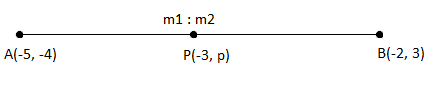Here,

(x1, y1) = (-5, -4)

(x2, y2) = (-2, 3)

(x, y) = (-3, p)

Using the formula of internal division,

x – coordinate of P is

x = (m1x2 + m2x1)/(m1 + m2)

-3 = [m1(-2) + m2(-5)] / (m1 + m2)

-3(m1 + m2) = -2m1 – 5m2

-3m1 – 3m2 = -2m1 – 5m2

⇒ 5m2 – 3 m2 = 3m1 – 2m1

⇒ 2m2 = m1

⇒ m1/m2 = 2

⇒ m1 : m2 = 2 : 1

y – coordinate of P is

y = (m1y2 + m2y1)/(m1 + m2)

p = [2(3) + 1(-4)] / (2 + 1)

= (6 – 4)/3

= 2/3

Hence, the required ratio is 2 : 1 and the value of p = 2/3.

### RBSE Maths Chapter 9: Additional Important Questions and Solutions

Question 1: Distance of point (3, 4) from y-axis will be:

(a) 1

(b) 4

(c) 2

(d) 3

Solution:

We know that, distance of a point from the y-axis will be the x-coordinate of that point.

Given point is (3, 4).

Therefore, the distance from y-axis = 3 units

Question 2: Distance of point (5, -2) from x-axis will be:

(a) 5

(b) 2

(c) 3

(d) 4

Solution:

We know that, distance of a point from the x-axis will be the y-coordinate of that point.

Given point is (5, -2).

Therefore, the distance from x-axis = 2 units

Question 3: Distance between points (0, 3) and (-2, 0) will be:

(a) √14

(b) √15

(c) √13

(d) √5

Solution:

Distance formula to find the distance between two points (x1, y1) and (x2, y2) is:

$$\begin{array}{l}d=\sqrt{(x_2-x_1)^2+(y_2-y_1)^2}\end{array}$$

Distance between (0, 3) and (-2, 0) is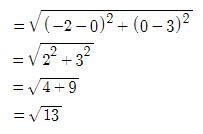Question 4:Quadrilateral having vertices (-1, 1), (0, -3), (5, 2) and (4, 6) will be:

(a) Square

(b) Rectangle

(c) Rhombus

(d) Parallelogram

Solution:

Let the given points be:

A = (-1, 1)

B = (0, -3)

C = (5, 2)

D = (4, 6)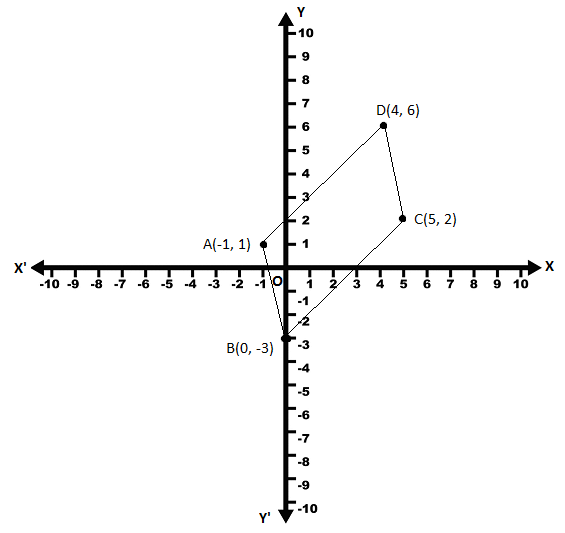After plotting the given points, the obtained figure is a parallelogram as shown above.

Question 5: Point equidistant from (0, 0), (2, 0) and (0, 2) is

(a) (1, 2)

(b) (2, 1)

(c) (2, 2)

(d) (1, 1)

Solution:

Let P(x, y) be the point which is equidistant from O(0, 0), A(2, 0) and B(0, 2).

PO = PA = PB

⇒ PO2 = PA2 = PB2

Now,

PO2 = PA2

(0 – x)2 + (0 – y)2 = (2 – x)2 + (0 – y)2

x2 + y2 = 4 + x2 – 4x + y2

⇒ 4x = 4

⇒ x = 4/4 = 1

PO2 = PB2

(0 – x)2 + (0 – y)2 = (0 – x)2 + (2 – y)2

x2 + y2 = x2 + 4 + y2 – 4y

⇒ 4y = 4

⇒ y = 4/4 = 1

Hence, the required point is (1, 1).

Question 6: P divides the line segment which joins points (5, 0) and (0, 4) in the ratio 2 : 3 internally. Coordinates of P are:

(a) (3, 8/5)

(b) (1, 4/5)

(c) (5/2, 3/4)

(d) (2, 12/5)

Solution:

Let P(x, y) divide the line segment joining the points A(5, 0) and B(0, 4) internally in the ratio 2 : 3.

Here,

Here,

(x1, y1) = (5, 0)

(x2, y2) = (0, 4)

m1 : m2 = 2 : 3

Using the formula of internal division,

x = (m1x2 + m2x1)/(m1 + m2)

= [2(0) + 3(5)]/(2 + 3)

= (0 + 15)/5

= 15/5

= 3

y = (m1y2 + m2y1)/(m1 + m2)

= [2(4) + 3(0)]/(2 + 3)

= (8 + 0)/5

= 8/5

Hence, the coordinates of the required point is P(3, 8/5).

Question 7: If distance between points (3, a) and (4, 1) is √10, then a will be

(a) 3, -1

(b) 2, -2

(c) 4, -2

(d) 5, -3

Solution:

Let the given points be:

A = (3, a)

B = (4, 1)

According to the given,

AB = √10

Squaring on both sides,

AB2 = (√10)2

(4 – 3)2 + (1 – a)2 = 10

(1)2 + 1 + a2 – 2a = 10

a2 – 2a + 1 + 1 – 10 = 0

a2 – 2a – 8 = 0

a2 – 4a + 2a – 8 = 0

a(a – 4) + 2(a – 4) = 0

(a + 2)(a – 4) = 0

a + 2 = 0, a – 4 = 0

a = -2, a = 4

Question 8: If point (x, y) is at equidistant from (2, 1) and (1, -2), then the true statement is:

(a) x + 3y = 0

(b) 3x + y = 0

(c) x + 2y = 0

(d) 2y + 3x = 0

Solution:

Let the given points be:

P = (x, y)

A = (2, 1)

B = (1, -2)

According to the given,

PA = PB

⇒ PA2 = PB2

⇒ (2 – x)2 + (1 – y)2 = (1 – x)2 + (-2 – y)2

⇒ 4 + x2 – 4x + 1 + y2 – 2y = 1 + x2 – 2x + 4 + y2 + 4y

⇒ 5 – 4x – 2y = 5 – 2x + 4y

⇒ -2x + 4y + 4x + 2y = 0

⇒ 2x + 6y = 0

⇒ 2(x + 3y) = 0

⇒ x + 3y = 0

Hence, the required relation is x + 3y = 0

Question 9: Find the type of quadrilateral, if its vertices are (1, 4), (-5, 4), (-5, -3) and (1, -3).

Solution:

Let the given points be:

A = (1, 4)

B = (-5, 4)

C = (-5, -3)

D = (1, -3)

Using the distance formula,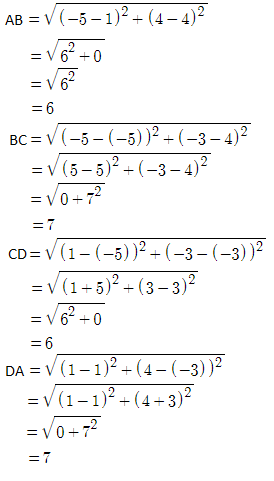From this, the opposite sides of a quadrilateral are equal.

Diagonals are AC and BD.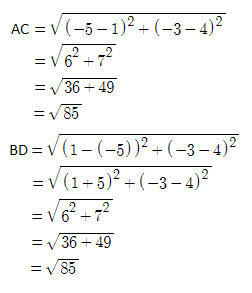AC = BD

Therefore, the given points are the vertices of a rectangle.

Question 10: Find the ratio in which the point (3, 4) divides the line segment which joins points (1, 2) and (6, 7).

Solution:

Let P(3, 4) be the point which divides the line segment joining the points A(1, 2) and B(6, 7) internally in the ratio k : 1.

Here,

(x1, y1) = (1, 2)

(x2, y2) = (6, 7)

m1 : m2 = k : 1

Using section formula,

x-coordinate of P is

x = (m1x2 + m2x1)/(m1 + m2)

3 = [k(6) + 1(1)] / (k + 1)

⇒ 3(k + 1) = 6k + 1

⇒ 3k + 3 = 6k + 1

⇒ 6k – 3k = 3 – 1

⇒ 3k = 2

⇒ k = ⅔

⇒ k/1 = 2/3

Therefore, the required ratio is 2 : 3.

Question 11: Opposite vertices of any square are (5, -4) and (-3, 2), then find the length of diagonal.

Solution:

Given,

Opposite vertices of a square are (5, -4) and (-3, 2).

Let A = (5, -4) and B = (-3, 2)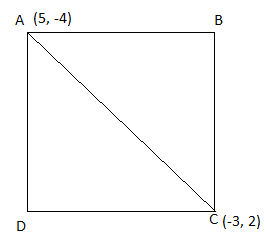Length of the diagonal = Distance between A and C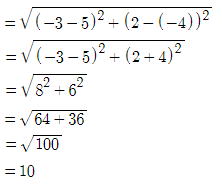Therefore, the length of the diagonal of the square is 10 units.

Question 12: If coordinates of one end and midpoint of a line segment are (4, 0) and (4, 1) respectively, then find the coordinates of the other end of the line segment.

Solution:

Let the given coordinates of one end and midpoint of segment are A(4, 0) and M(4, 1).

Let B(x, y) be the coordinates of the point on the other end of the segment.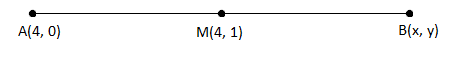M is the midpoint of AB.

Here,

(x1, y1) = (4, 0)

(x2, y2) = (x, y)

Using midpoint formula,

x-coordinate of the midpoint:

4 = (x1 + x2)/2

4 = (4 + x)/2

8 = 4 + x

⇒ x = 8 – 4 = 4

y-coordinate of the midpoint:

1 = (y1 + y2)/2

1 = (0 + y)/2

⇒ y = 2

Hence, the required coordinates are (4, 2).

Question 13: Find the distance of the point (1, 2) from the midpoint of the line segment which joins the points (6, 8) and (2, 4).

Solution:

Let P(x, y) be the midpoint of line joining the points A(6, 8) and B(2, 4)

Using the midpoint formula,

P(x, y) = [(6 + 2)/2, (8 + 4)/2]

= (8/2, 12/2)

= (4, 6)

P(x, y) = (4, 6)

Now, Q = (1, 2).

Distance between PQ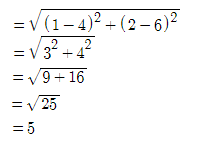Hence, the required distance is 5 units.

Question 14: If there are four points P(2, -1), Q(3, 4), R(-2, 3) and S(-3, -2) in a plane, then prove that PQRS is not a square but a rhombus.

Solution:

Given points are:

P(2, -1), Q(3, 4), R(-2, 3) and S(-3, -2)

Using the distance formula,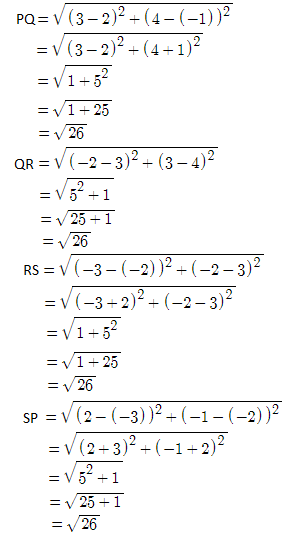Diagonals are PR and QS.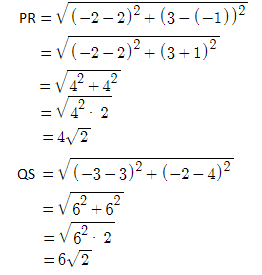Therefore, PQ = QR = RS = SP and PR ≠ QS

Hence, the given points are the vertices of a rhombus.

Question 15: If distance between points (x, 3) and (5, 7) is 5, then find the value of x.

Solution:

Let the given points be:

A = (x, 3)

B = (5, 7)

According to the given,

AB = 5

Squaring on both sides,

AB2 = 52

(5 – x)2 + (7 – 3)2 = 25

25 + x2 – 10x + (4)2 = 25

x2 – 10x + 16 = 0

x2 – 2x – 8x + 16 = 0

x(x – 2) – 8(x – 2) = 0

(x – 8)(x – 2) = 0

⇒ x – 8 = 0, x – 2 = 0

⇒ x = 8, x = 2